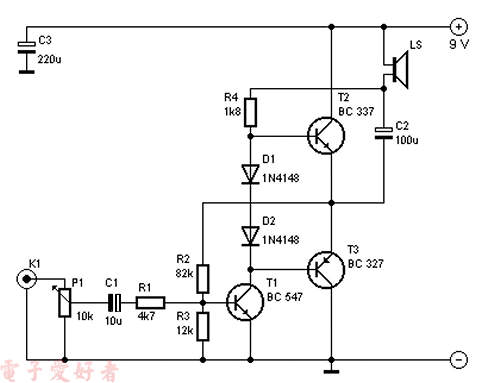﻿ 分立元件250mW的OTL放大器_音响_电子爱好者

# 分立元件250mW的OTL放大器R1 = 4,7 kΩ
R2 = 82 kΩ
R3 = 12 kΩ
R4 = 1,8 kΩ
P1 = 10 kΩ
C1 = 10 µF/10 V
C2 = 100 µF/10 V
C3 = 220 µF/10 V
D1, D2 = 1N4148
T1 = BC 547
T2 = BC 337
T3 = BC 327